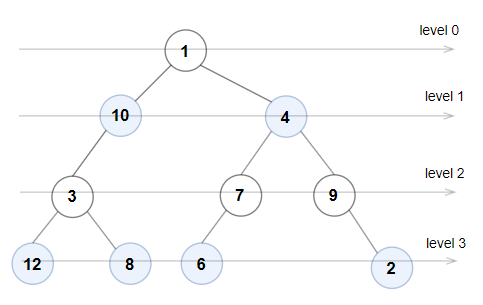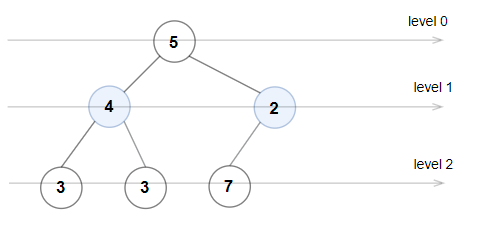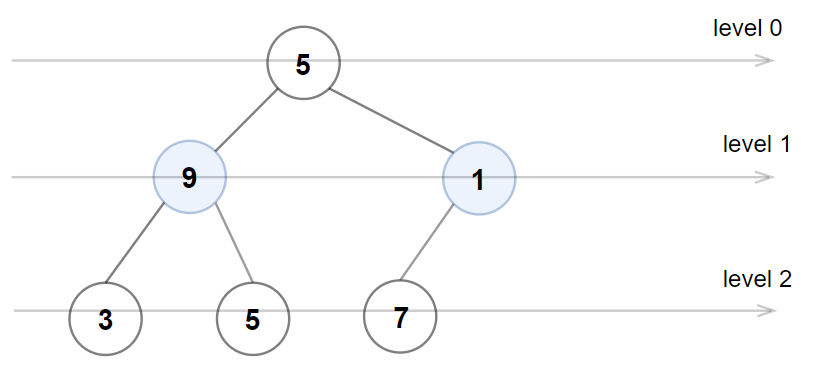##### Welcome to Subscribe On Youtube

Formatted question description: https://leetcode.ca/all/1609.html

# 1609. Even Odd Tree (Medium)

A binary tree is named Even-Odd if it meets the following conditions:

• The root of the binary tree is at level index 0, its children are at level index 1, their children are at level index 2, etc.
• For every even-indexed level, all nodes at the level have odd integer values in strictly increasing order (from left to right).
• For every odd-indexed level, all nodes at the level have even integer values in strictly decreasing order (from left to right).

Given the root of a binary tree, return true if the binary tree is Even-Odd, otherwise return false.

Example 1:Input: root = [1,10,4,3,null,7,9,12,8,6,null,null,2]
Output: true
Explanation: The node values on each level are:
Level 0: 
Level 1: [10,4]
Level 2: [3,7,9]
Level 3: [12,8,6,2]
Since levels 0 and 2 are all odd and increasing, and levels 1 and 3 are all even and decreasing, the tree is Even-Odd.


Example 2:Input: root = [5,4,2,3,3,7]
Output: false
Explanation: The node values on each level are:
Level 0: 
Level 1: [4,2]
Level 2: [3,3,7]
Node values in the level 2 must be in strictly increasing order, so the tree is not Even-Odd.


Example 3:Input: root = [5,9,1,3,5,7]
Output: false
Explanation: Node values in the level 1 should be even integers.


Example 4:

Input: root = 
Output: true


Example 5:

Input: root = [11,8,6,1,3,9,11,30,20,18,16,12,10,4,2,17]
Output: true


Constraints:

• The number of nodes in the tree is in the range [1, 105].
• 1 <= Node.val <= 106

Related Topics:
Tree

## Solution 1. Level-order traversal

// OJ: https://leetcode.com/problems/even-odd-tree/
// Time: O(N)
// Space: O(N)
class Solution {
public:
bool isEvenOddTree(TreeNode* root) {
queue<TreeNode*> q;
q.push(root);
int lv = 0;
while (q.size()) {
int cnt = q.size(), prev = lv % 2 == 0 ? 0 : INT_MAX;
while (cnt--) {
auto node = q.front();
q.pop();
if (lv % 2 == 0) {
if (node->val % 2 == 0 || node->val <= prev) return false;
} else {
if (node->val % 2 || node->val >= prev) return false;
}
prev = node->val;
if (node->left) q.push(node->left);
if (node->right) q.push(node->right);
}
++lv;
}
return true;
}
};


Java

• /**
* Definition for a binary tree node.
* public class TreeNode {
*     int val;
*     TreeNode left;
*     TreeNode right;
*     TreeNode() {}
*     TreeNode(int val) { this.val = val; }
*     TreeNode(int val, TreeNode left, TreeNode right) {
*         this.val = val;
*         this.left = left;
*         this.right = right;
*     }
* }
*/
class Solution {
public boolean isEvenOddTree(TreeNode root) {
if (root == null)
return false;
queue.offer(root);
int level = 0;
while (!queue.isEmpty()) {
int size = queue.size();
int prev = level % 2 == 0 ? Integer.MIN_VALUE : Integer.MAX_VALUE;
for (int i = 0; i < size; i++) {
TreeNode node = queue.poll();
int value = node.val;
if (level % 2 == value % 2)
return false;
if (level % 2 == 0 && value <= prev || level % 2 == 1 && value >= prev)
return false;
prev = value;
TreeNode left = node.left, right = node.right;
if (left != null)
queue.offer(left);
if (right != null)
queue.offer(right);
}
level++;
}
return true;
}
}

• // OJ: https://leetcode.com/problems/even-odd-tree/
// Time: O(N)
// Space: O(N)
class Solution {
public:
bool isEvenOddTree(TreeNode* root) {
queue<TreeNode*> q;
q.push(root);
int lv = 0;
while (q.size()) {
int cnt = q.size(), prev = lv % 2 == 0 ? 0 : INT_MAX;
while (cnt--) {
auto node = q.front();
q.pop();
if (lv % 2 == 0) {
if (node->val % 2 == 0 || node->val <= prev) return false;
} else {
if (node->val % 2 || node->val >= prev) return false;
}
prev = node->val;
if (node->left) q.push(node->left);
if (node->right) q.push(node->right);
}
++lv;
}
return true;
}
};

• # Definition for a binary tree node.
# class TreeNode:
#     def __init__(self, val=0, left=None, right=None):
#         self.val = val
#         self.left = left
#         self.right = right
class Solution:
def isEvenOddTree(self, root: Optional[TreeNode]) -> bool:
even = 1
q = deque([root])
while q:
prev = 0 if even else inf
for _ in range(len(q)):
root = q.popleft()
if even and (root.val % 2 == 0 or prev >= root.val):
return False
if not even and (root.val % 2 == 1 or prev <= root.val):
return False
prev = root.val
if root.left:
q.append(root.left)
if root.right:
q.append(root.right)
even ^= 1
return True

############

# 1609. Even Odd Tree
# https://leetcode.com/problems/even-odd-tree/

# Definition for a binary tree node.
# class TreeNode:
#     def __init__(self, val=0, left=None, right=None):
#         self.val = val
#         self.left = left
#         self.right = right
class Solution:
def isEvenOddTree(self, root: TreeNode) -> bool:
if not root: return False

q = deque()
q.append(root)
isEven = True
while q:

n = len(q)
check = []
prev = None
while n:
node = q.popleft()
val = node.val
if isEven:
if val % 2 == 0 or prev and val <= prev:
return False
else:
if val % 2 or prev and val >= prev:
return False

prev = val

for t in (node.left,node.right):
if t:
q.append(t)

n -= 1

isEven = not isEven

return True